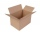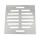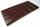# Squares

A square of 12 dm perimeter is cut smaller square, whose side is the third side of the square. What is the area of the rest?

S =  8 dm2

### Step-by-step explanation:Did you find an error or inaccuracy? Feel free to write us. Thank you!#### You need to know the following knowledge to solve this word math problem:

We encourage you to watch this tutorial video on this math problem:

## Related math problems and questions:

• Cardboard boxPeter had square cardboard. The length of the pages was an integer in decimetres. He cut four squares with a side of 3 dm from the corners and made a box out of it, which fit exactly 108 cubes with an edge 1 dm long. Julia cut four squares with a side of
• Square plateThe locksmith cut a square metal plate with a sidelong 6 dm into two identical rectangular openings with 2.5 dm and 2 dm dimensions. Calculate the remainder of the square plate.
• Largest squaresHow many of the largest square sheets did the plumber cut the honeycomb from 16 dm and 96 dm?
• Glass panelA rectangular glass panel with dimensions of 72 cm and 96 cm will cut the glazier on the largest square possible. What is the length of the side of each square? How many squares does the glazier cut?
• PlumberThe plumber had to cut the metal strip with dimensions 380 cm and 60 cm to the largest squares to no waste. Calculate the length of the sides of a square. How many squares cut it?
• Octagon from rectangleFrom tablecloth rectangular shape with dimensions of 4 dm and 8 dm we cuts down the corners in the shape of isosceles triangles. It thus formed an octagon with area 26 dm2. How many dm2 we cuts down?
• Paper squaresThe paper rectangle measuring 69 cm and 46 cm should be cut into as many squares as possible. Calculate the lengths of squares and their number.
• RectangleThe rectangle area is 182 dm2, its base is 14 dm. How long is the other side? Calculate its perimeter.
• Parallelogram - sideCalculate the length of the side of a parallelogram whose area is 175 dm2 and height to that side is 24.2 dm long.
• Two squaresTwo squares whose sides are in the ratio 5:2 have a sum of its perimeters 73 cm. Calculate the sum of the area of these two squares.
• ChocholateTable of chocolate is divided into squares on its surface. Lengthwise has 15 squares and widthwise 19 squares. We must chocolate broke into individual squares. How many times have we broke it to get only individual squares? It is not permitted to break se
• Square metal sheetWe cut out four squares of 300 mm side from a square sheet metal plate with a side of 0,7 m. Express the fraction and the percentage of waste from the square metal sheet.
• Squares ratioThe first square has a side length of a = 6 cm. The second square has a circumference of 6 dm. Calculate the proportions of the perimeters and the proportions of the contents of these squares? (Write the ratio in the basic form). (Perimeter = 4 * a, conte
• Recursion squaresIn the square, ABCD has inscribed a square so that its vertices lie at the centers of the sides of the square ABCD. The procedure of inscribing the square is repeated this way. The side length of the square ABCD is a = 22 cm. Calculate: a) the sum of peri
• MO-Z5-3-66 tilesThe picture shows a square tiles with side 10 dm which is composed of four identical small rectangles and squares. Circumference of small square is five times smaller than the circumference of the entire tile. Determine the dimensions of the rectangle.
• Square glassWhat is the area of the square window glass with the side 4 dm? Do you know how many cm2 is it?
• Area of squareCalculate the content area of the square whose perimeter is 24 dm.